# Norm-residue symbol

(diff) ← Older revision | Latest revision (diff) | Newer revision → (diff)

norm residue, Hilbert symbol

A function that associates with an ordered pair of elementsof the multiplicative group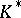of a local fieldan element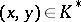that is an-th root of unity. This function can be defined as follows. Let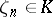be a primitive-th root of unity. The maximal Abelian extensionofwith Galois group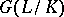of exponentis obtained by adjoining tothe rootsfor all. On the other hand, there is a canonical isomorphism (the fundamental isomorphism of local class field theory)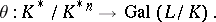The norm residue of the pair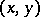is defined by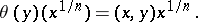D. Hilbert introduced the concept of a norm-residue symbol in the special case of quadratic fields with. In  there is an explicit definition of the norm residue using only local class field theory.

Properties of the symbol:

1) bilinearity:,;

2) skew-symmetry:;

3) non-degeneracy: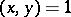for all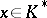implies;for allimplies;

4) if, then;

5) ifis an automorphism of, then6) let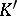be a finite extension of,and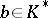. Then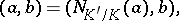where on the left-hand side the norm-residue symbol is regarded forand on the right-hand side that for, and where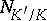is the norm map frominto;

7)implies thatis a norm in the extension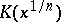. (This explains the name of the symbol.)

The functioninduces a non-degenerate bilinear pairingwhere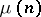is the group of roots of unity generated by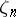. Let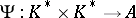be a mapping into some Abelian groupsatisfying 1), 4) and the condition of continuity: For any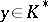the setis closed in. The norm-residue symbol has the following universal property : Ifis the number of roots of unity in, then there exists a homomorphism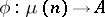such that for any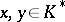,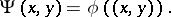This property can serve as a basic axiomatic definition of the norm-residue symbol.

Ifis a global field andis the completion ofrelative to a place, then by the norm-residue symbol one also means the functiondefined over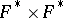that is obtained by composition of the (local) norm-residue symbolwith the natural imbedding.

Often the norm-residue symbol is defined as an automorphismof the maximal Abelian extension ofcorresponding to an elementby local class field theory.

How to Cite This Entry:
Norm-residue symbol. Encyclopedia of Mathematics. URL: http://encyclopediaofmath.org/index.php?title=Norm-residue_symbol&oldid=12271
This article was adapted from an original article by L.V. Kuz'min (originator), which appeared in Encyclopedia of Mathematics - ISBN 1402006098. See original article